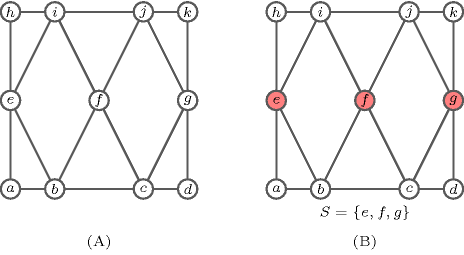### 3.7.90. Domination

A constraint that can be used for expressing directly the fact that we search for a dominating set in an undirected graph. Given an undirected graph $G=\left(V,E\right)$ where $V$ is a finite set of vertices and $E$ a finite set of unordered pairs of distinct elements from $V$, a set $S$ is a dominating set if for every vertex $u\in V-S$ there exists a vertex $v\in S$ such that $u$ is adjacent to $v$. Part (A) of Figure 3.7.26 gives an undirected graph $G$, while part (B) depicts a dominating set $S=\left\{e,f,g\right\}$ in $G$.

##### Figure 3.7.26. (A) A graph and (B) one of its dominating set $S=\left\{e,f,g\right\}$